•matlab
• 关于可见光通信信道建模的有关对数正态pdf的MATLAB代码。
• Matlab R2014b以来,事情变得非常简单.不需要文件交换的附加功能,只需要一些undocumented features.基本思想是获取标记的隐藏句柄并应用值< 1为EdgeColorData中的最后一个值,以实现所需的透明度. 开始了：%// ...

自Matlab R2014b以来,事情变得非常简单.不需要文件交换的附加功能,只需要一些

undocumented features.基本思想是获取标记的隐藏句柄并应用值< 1为EdgeColorData中的最后一个值,以实现所需的透明度. 开始了：

%// example data

x = linspace(0,3*pi,200);

y = cos(x) + rand(1,200);

%// plot scatter, get handle

h = scatter(x,y);

drawnow; %// important

%// get marker handle

hMarkers = h.MarkerHandle;

%// get current edge and face color

edgeColor = hMarkers.EdgeColorData

faceColor = hMarkers.FaceColorData

%// set face color to the same as edge color

faceColor = edgeColor;

%// opacity

opa = 0.3;

%// set marker edge and face color

hMarkers.EdgeColorData = uint8( [edgeColor(1:3); 255*opa] );

hMarkers.FaceColorData = uint8( [faceColor(1:3); 255*opa] );展开全文• MATLAB 二维图形 直方 扇形 柱状

本实验取材于中南大学《MATLAB与科学计算》
今天学习主要有两种内容，第一、对数坐标图，第二、统计图
一、对数坐标图

semilogx(x1,y1,选项1,x2,y2,选项2,...)
semilogy(x1,y1,选项1,x2,y2,选项2,....)
loglog(x1,y1,选项1,x2,y2,选项2,...)

例子：绘出1/x的函数图像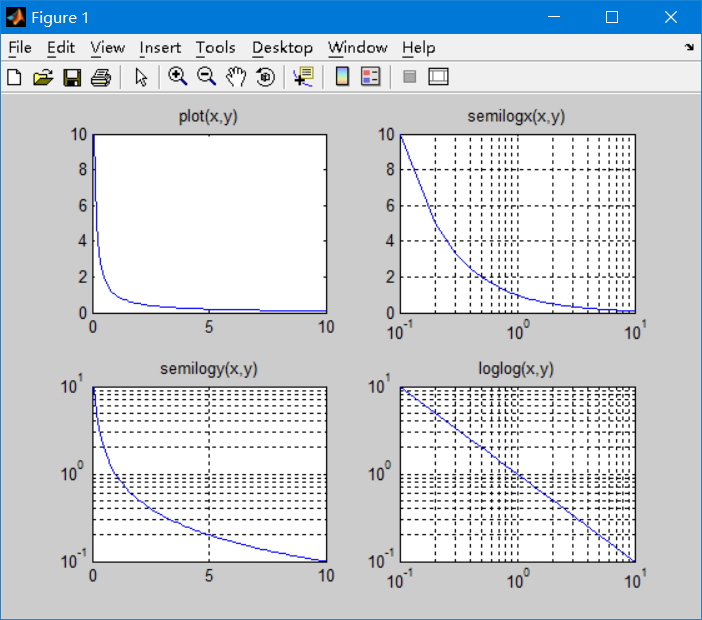代码

x=0:0.1:10;
y=1./x;
subplot(2,2,1);
plot(x,y)
title('plot(x,y)');
subplot(2,2,2);
semilogx(x,y)
title('semilogx(x,y)');
grid on
subplot(2,2,3);
semilogy(x,y);
title('semilogy(x,y)');
grid on
subplot(2,2,4);
loglog(x,y)
title('loglog(x,y)');
grid on

总结：其实就是对数的转换，你想转换x那就semilogx，如果想转换y那就semilogy，如果想都转换一样，那就是loglog

二、统计图
1、条形图

格式

bar(y,style)

ps:style可以有两类，grouped簇状分组 stacked 为堆积分组

代码：

hold off
x=[2015,2016,2017];
y=[68,80,115,98,102;75,88,102,99,110;81,86,125,105,115];
bar(x,y)
title('Group')

图片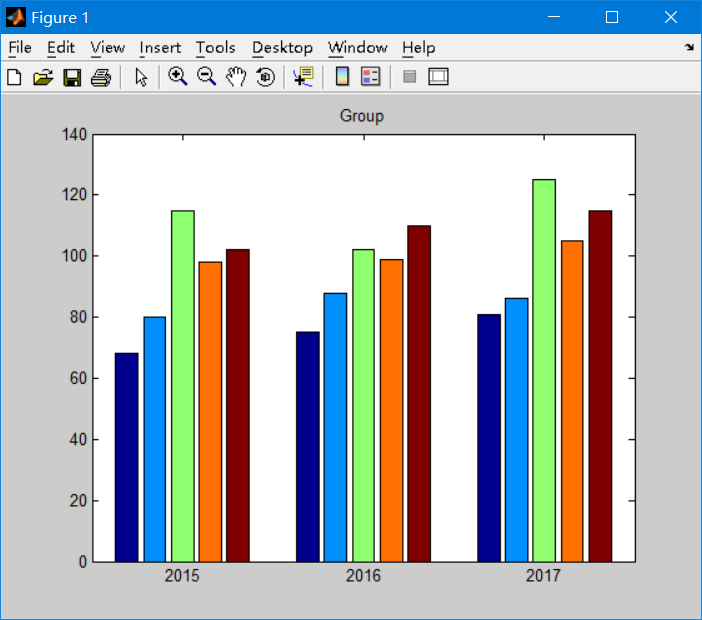2、直方图
格式

hist(x,y)

代码

>> y=randn(500,1);
>> subplot(2,1,1);
>> hist(y);
>> title('高斯分布直方图');
>> subplot(2,1,2);
>> x=-3:0.2:3;
>> hist(y,x);
>> title('指定区间中心点的直方图')
>>

图片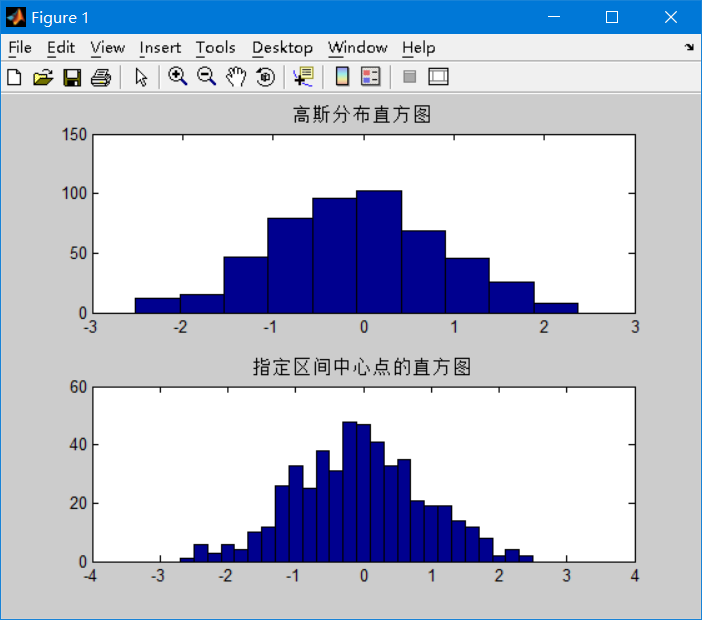3、饼图
格式：

pie(x,y)

代码

score=[5,17,23,9,4];
ex=[0,0,0,0,1];
hold
pie(score,ex)
legend('优秀','良好','中等','及格','不及格','location','eastoutside')

图片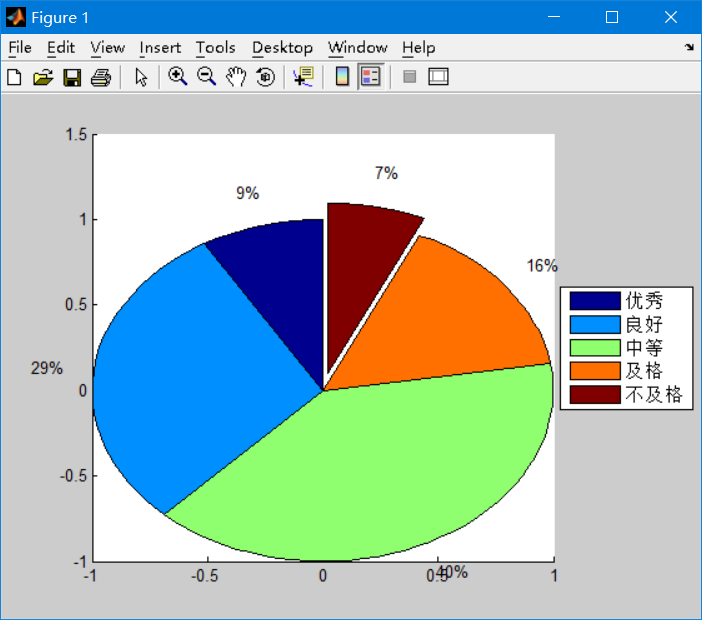展开全文• 在Mathworks网站上有一个讨论，有人提供了一个函数来做对数彩条。编辑：复制和粘贴的链接代码function cbar = colorbar_log(my_clim)%COLORBAR_LOG Apply log10 scaling to pseudocolor axis% and display colorbar ...

在Mathworks网站上有一个讨论，有人提供了一个函数来做对数彩条。

编辑：复制和粘贴的链接代码

function cbar = colorbar_log(my_clim)

%COLORBAR_LOG Apply log10 scaling to pseudocolor axis

% and display colorbar COLORBAR_LOG(V), where V is the

% two element vector [cmin cmax], sets manual, logarithmic

% scaling of pseudocolor for the SURFACE and PATCH

% objects. cmin and cmax should be specified on a LINEAR

% scale, and are assigned to the first and last colors in

% the current colormap. A logarithmic scale is computed,

% then applied, and a colorbar is appended to the current

% axis.

%

% Written by Matthew Crema - 7/2007

% Trick MATLAB by first applying pseudocolor axis

% on a linear scale

caxis(my_clim)

% Create a colorbar with log scale

cbar = colorbar('Yscale', 'log');

% Now change the pseudocolor axis to a log scale.

caxis(log10(my_clim));

% Do not issue the COLORBAR command again! If you want to

% change things, issue COLORBAR_LOG again.

展开全文• 对数变换是matlab图像处理技术中增强图像的一种预处理方法 ，当希望对图像的低亮度区有较大的扩展而对高亮度区压缩时，可采用此种变换。
• 将一组数，按照给定的规模进行分区。如：将1000个节点,划分到71个社区，每个社区内的元素数都在10-20之间。
• 选择一个图像并应用 log_expT.m ti 你可以得到对数变换和对数变换图像的相应指数变换。 以便我们可以看到原始图像和指数图像之间的差异。matlab
• 教你用matlab对数坐标！在很多工程问题中，通过对数据进行对数转换可以更清晰地看出数据的某些特征，在对数坐标系中描绘数据点的曲线，可以直接地表现对数转换。对数转换有双对数坐标转换和单轴对数坐标转换两种。...

原标题：大神经验！教你用matlab画对数坐标！

在很多工程问题中，通过对数据进行对数转换可以更清晰地看出数据的某些特征，在对数坐标系中描绘数据点的曲线，可以直接地表现对数转换。

对数转换有双对数坐标转换和单轴对数坐标转换两种。用loglog函数可以实现双对数坐标转换，用semilogx和semilogy函数可以实现单轴对数坐标转换。

loglog(Y) 表示 x、y坐标都是对数坐标系

semilogx(Y) 表示 x坐标轴是对数坐标系

semilogy(…) 表示y坐标轴是对数坐标系

plotyy 有两个y坐标轴，一个在左边，一个在右边

例1：

用方形标记创建一个简单的loglog.

解: 输入命令

x=logspace(-1,2);

loglog(x,exp(x),'-s')

grid on %标注格栅

所制图形为: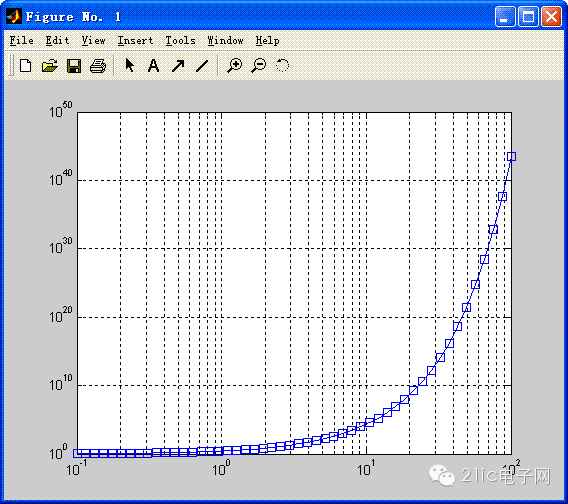例2：

创建一个简单的半对数坐标图.

解 输入命令:

x=0:.1:10;

semilogy(x,10.^x)

所制图形为：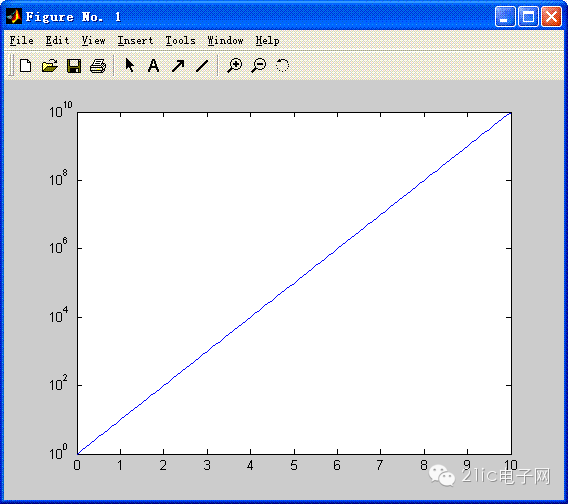例3：

绘制y=x^3的函数图、对数坐标图、半对数坐标图.

解:在窗口中输入：

x=[1:1:100];

subplot(2,3,1);

plot(x,x.^3);

grid on;

title 'plot-y=x^3';

subplot(2,3,2);

loglog(x,x.^3);

grid on;

title 'loglog-logy=3logx';

subplot(2,3,3);

plotyy(x,x.^3,x,x);

grid on;

title 'plotyy-y=x^3,logy=3logx';

subplot(2,3,4);

semilogx(x,x.^3);

grid on;

title 'semilogx-y=3logx';

subplot(2,3,5);

semilogy(x,x.^3);

grid on;

title 'semilogy-logy=x^3';

所制图形为：

责任编辑：

展开全文• 文档中包含网易云课堂MATLAB教程中 冒号区间裁剪，冒号等差序列，删除整行整列的操作，简单易学，内容详细。MATLAB 数组
• matlab对数函数怎么表示

千次阅读 2021-04-23 23:11:19
MATLAB 中如何输入 对数函数趁阳光正好，趁微风不噪。趁繁花还未开至荼蘼，趁现在还年轻。还可以走很长很长的路，还能诉说很深很深的思念。去寻找那些曾出现在梦境中的路径山峦与田野吧。在MATLAB中输入对数函数主要...
• MATLAB画对数坐标

万次阅读 2018-06-15 22:31:55
在很多工程问题中，通过对数据进行对数转换可以更清晰地看出数据的某些特征，在对数坐标系中描绘数据点的曲线，可以直接地表现对数转换。对数转换有双对数坐标转换和单轴对数坐标转换两种。用loglog函数可以实现双...
• MATLAB对数刻度绘图 （不等间距坐标绘图） 主要概括 这两天师兄算了一个风速，有了采样时间和风速大小，让我帮忙绘制风速的功率密度谱函数，到最后看到不等比的坐标轴怎么画，最后搜出了，写在这边以供查找。 原 ...MATLAB
• Matlab的对数

2021-04-23 21:51:31
但是在matlab中，log默认为e的对数，而如果需要其他的对数，则是log10，log2等等；例如：log10(10)=1等等。rand - Uniformly distributed pseudorandom numbersThis MATLAB function returns a pseudorandom s...
• 包含对正态分布和对数正态分布的直方数据的拟合。 虽然仍然需要拟合工具箱，但参数的调整方式使 Matlab 可以实际拟合值（例如非常小的 x 值）！ 函数返回 mu 和 sigma 参数、缩放因子（对于非单位分布）和拟合...matlab
• 在很多工程问题中，通过对数据进行对数转换可以更清晰地看出数据的某些特征，在对数坐标系中描绘数据点的曲线，可以直接地表现对数转换。对数转换有双对数坐标转换和单轴对数坐标转换两种。用loglog函数可以实现双...
• matlab对数计算方法

千次阅读 2020-07-10 11:58:39windows
• matlab对数坐标的使用

万次阅读 2018-05-24 10:44:40
画图时有时不想使用自然坐标（线性刻度），而需要使用对数坐标，此处给出几种方法：1使用ezplot画图时，坐标用对数坐标。 ezplot默认横坐标从-2pi到2pi 举例：以y=x为例 a) 其中一方为对数坐标，另一方为线性坐标 ...对数坐标 matlab
• 本教程分享：《matlab对数函数》，MATLAB 中如何输入 对数函数方法/步骤1、自然数对数 log(x)我们在MATLAB主窗口中输入a1=log(2.7183)，回车，我们可以看到a1近似为1，e约等于2.7183,2、以2为底数的对数 log2(x)我们...
• 后来看了这个例子发现不是X维数的原因，其原因是对数函数ln(x)的x值要大于0，我的数据算出来均为负数，所以才出现了这样的情况。所以我将x改为绝对值的形式，就避免了这种情况。并且关于optimset函数，是一个优化...
• 计算对数似然相似度 clc,clear; %计算对数似然相似度 K=[1 2 3; 1 2 3; 1 2 3]; N=sum(sum(K)); %计算行熵 rowEntropy=[K(1,1)*log(K(1,1)/sum(K(1,:))),K(1,2)*log(K(1,2)/sum(K(1,:))),K(2,1)*log(K(2,1)/sum(K...matlab
• 得到数据和概率分布之前用水晶球软件算第一步很简单，但是我的其他几组数据都不足十个，没办法只好用MATLAB代替。那么问题来了：问题1 我知道怎么判断正态分布、指数分布等，求一个函数能求出一组数据的对数分布函...
• -------------------------------------------------- ---------- 目的：执行对数线性化。 -------------------------------------------------- ---------- 语法：result = LogLin( VarEndoNames, VarExoNames, ...matlab
• 代码亲测可用，直接调用comp_llr函数，格式是comp_llr(cleanFile, enhancedFile)，分别是干净语音，带噪语音
• matlab对数坐标及拟合使用的函数及使用方法
• 此代码演示了如何使用Log Transform进行图像增强matlab
• LOGZPLOT 是一种使用对数刻度 z 轴和对数刻度着色创建表面的简单方法。 LOGZPLOT 使用 SURF、MESH、IMAGE、PCOLOR、TRISURF 或 TRIMESH 创建绘图，然后应用对数变换。 如果在没有数据输入的情况下调用，LOGZPLOT ...matlab
• matlab画对数坐标图

千次阅读 2019-12-24 22:22:03
close all; x = [256 512 1024 2048 4096 8192]; y1 = [0.017360, 0.043902, 0.185930, 0.787196, 3.266647, 45.045046] y2 = [0.000510, 0.000625, 0.000573, 0.000602, 0.000635, 0.000714] semilogy(x,y1,'b*-',....
• 对数函数运算法则是什么呢？●中文名对数函数外文名LogarithmicFunction别称对函数表达式ylogax(a0a≠1)提出者约翰·纳皮尔提出时间16世纪末应用学科数学适用领域范围解析几何适用领域范围代数学自然科学函数最值无......

matlab对数图matlab 订阅